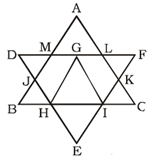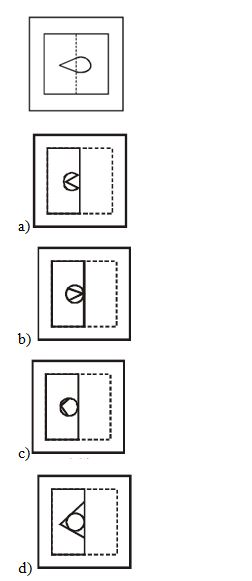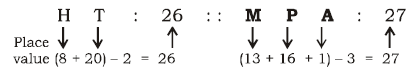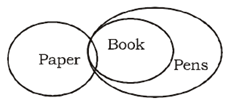# SSC CGL 2018 Practice Test Papers | Reasoning Ability (Day-36)

Dear Aspirants, Here we have given the Important SSC CGL Exam 2018 Practice Test Papers. Candidates those who are preparing for SSC CGL 2018 can practice these questions to get more confidence to Crack SSC CGL 2018 Examination.

[WpProQuiz 3235]

Click “Start Quiz” to attend these Questions and view Explanation

1) Select the related letters/ word/ number from the given alternatives.

a) Cabbage

b) Carrot

c) Potato

d) Beetroot

2) Select the related letters/ word/ number from the given alternatives.

HT : 26 :: ? : 27

a) YZA

b) MPA

c) LMB

d) XYB

3) How many triangles are there in the following figures?a) 10

b) 11

c) 12

d) 13

4) If the time in the mirror image of a clock is 4 hours 20 minutes, then what will be theactual time in this situation?

a) 7 : 30

b) 7 : 20

c) 7 : 40

d) 7 : 00

Direction: (5-6) one or two statements are given followed by some conclusions. You have to consider the two statements to be true even if they seem to be at variance from commonly known facts. You have to decide which of the given conclusions, if any, follow from the given statements.

5) Statement:

Many drug addicts were arrested by police.

Conclusions:

Taking drug is a crime.

Many people take drugs regularly.

a) Only I conclusion follows.

b) Only II conclusion follows.

c) Both follow.

d) None of these

6) Statements:

Some papers are books.

All books are pens.

Conclusions:

Some pens are paper.

Some pens are book.

a) Only I conclusion follows.

b) Only II conclusion follows.

c) Both follow.

d) None of these

7) In the following questions given format is made on a transparent page and folded along the dotted line, then how will it be visible ?a)

b)

c)

d)

8) From the options given below, which option is according to the dictionary?

i) Peacock

ii) Penguin

iii) Pesticide

iv) Parrot

a) iv, iii, ii, i

b) iv, i, ii, iii

c) ii, ii, iv, i

d) i, ii, iv, iii

9) A word is represented by only one set of numbers as given in any one of the alternatives. The sets of numbers given in any one of the alternatives. The sets of numbers given in the alternatives are represented by two classes of alphabets as in two matrices given below. The columns and rows of matrix I are numbered from 0 to 4 And that matrix II are numbered from 5 to 9. A letter from these matrices can be represented first by its row and next by its column. Eg: A can be represented by21, 44, 76…Similarly you have to identify the set for the word ‘DUCK’

Matrix I

 0 1 2 3 4 0 D Z T P U 1 S Q M R Y 2 B A C S V 3 T W D G F 4 O L K U A

Matrix II

 5 6 7 8 9 5 U E Y X R 6 D U O U K 7 Y A K C U 8 R D T Y J 9 V N M I D

a) 99, 04, 22, 34

b) 86, 68, 78, 42

c) 96. 46, 32, 11

d) 99, 78, 05, 34

10) If \$ means ‘added to’, # means ‘multiplied by’, % means ‘divided by’ and & means ‘difference of’ then what is the value of 7 \$ 55 % 11 # 9 & 45.

a) 9

b) 7

c) -7

d) -9

Except option a), all are grown under soil.There are 11 triangles in the given figure: – ABC, DEF, GHI, AML, LFK, KCI, IEH, DGH, GFI, BHJ and MDJ.

Reflection time = 4 : 20

Actual time = 11 : 60 – 4 : 20 = 7 : 40

Direction (5-6)iv) Parrot

i) Peacock

ii) Penguin

iii) Pesticide